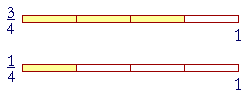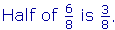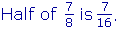S k i l l
i n
A R I T H M E T I C

Lesson 27  Section 2

# Parts of Fractions

Back to Section 1

Example 1 .   A recipe calls for 6cups of flour, and you are going to make a third of the recipe.  How much flour will you use?

Answer.  A third of 6 cups is 2 cups.  But how much is a third of?A third ofis.  Simply take a third of the numerator.

A third of  6cups is 2cups.

But say that the recipe calls for 6cups of flour. Since the numerator 1 does not have a third part, how will you take a third of?If we divide eachinto thirds, then the whole 1 will be in three times as many parts. A third ofis.

To take a third of a fraction, simply multiply the denominator by 3.

A third of  6cups is 2cups.

There are two ways, then, to take a part of a fraction.

 4. How can we take a part of a fraction?Take that part of the numerator -- if the numerator has that part.If the numerator does not have that part,multiply the denominator by the cardinal number that corresponds to the part. That is, to take half, multiply the denominator by 2; to take a third, multiply by 3; and so on.Example 2.

 Half of 67 is 37 . Take half of the numerator. A third of 67 is 27 . Take a third of the numerator. A third of 47 is 4 21 . 4 does not have a third part.  Therefore multiply the denominator by 3.
 Example 3.   How much is a fifth of 12 ?
 Answer. 1 10 .   Multiply 2 by 5.
 Example 4.   How much is half of 1 10 ?
 Answer. 1 20 .  Multiply 10 by 2.
 Example 5. 18 is which part of 12 ?
 Answer.   Since 8 is 4 × 2, 18 is the fourth part, or one quarter, of 12 .
 Example 6. 1 16 is which part of 18 ?   What ratio has 1 32 to 18 ?
 Answer. 1 16 is half of 18 .  16 = 2 × 8. 1 32 is a fourth of 18 .  32 = 4 × 8.
 Example 7.  Percent.   Since 18 is half of 14 (8 = 2 × 4),
 and since 14 is equal to 25%, then what percent is 18 ?

Answer.  Half of 25%, which is 12½%.  (Lesson 16, Example 4.)

Now, each eighth will be another 12½%.

 28 = 14 = 12½% + 12½% = 25%.
 38 = 25% + 12½% = 37½%.
 48 = 12 = 50%.
 58 = 50% + 12½% = 62½%.
 68 = 34 = 75%.
 78 = 75% + 12½% = 87½%.

See Problem 15.

 Example 8. 25 is larger than 2 25 . (Lesson 23, Question 1.)  How many

times larger?

Answer.  Five times larger.  Because 25 is 5 × 5.

Example 9.   The following problem appeared in a recent textbook:

 15 is 12 of what number?

The writer no doubt intend it to be translated as

 12 times what number is 15 ?

-- thus making it a division problem:

 15 ÷ 12 .

However, if we make verbal sense of the problem --

1-fifth is half of what number?

-- then the answer is obvious.  Just as 1 apple is half of 2 apples, so

1-fifth is half of 2-fifths.

 Example 10. 37 is half of what number?
 Answer. 37 is half of 67 .
 Example 11. 37 is a third of what number?
 Answer. 37 is a third of 97 , which is 1 27 .

Example 12.   To make an orange dye, 3 parts of red dye are mixed with 2 parts of yellow dye.  To make a green dye, 2 parts of blue dye are mixed with 1 part of yellow dye.  If equal amounts of orange and green are mixed, what fraction of the new mixture is yellow dye?

Solution.   For convenience, let

O = Orange dye,  R = Red dye,  Y = Yellow dye,

G = Green dye,  B = Blue dye.

Now, the orange dye consists of a total of five parts:  3 parts red and 2 parts yellow.  That is,

 O = 35 R + 25 Y.

The green dye consists of three parts:  2 parts blue and 1 part yellow.

 G = 23 B + 13 Y.

To add equal amounts of O and G, we may simply add half of each.   Therefore, half of O is

 O = 3 10 R + 15 Y.

And half of G is

 O = 13 B + 16 Y.

Upon adding those equal parts of O and G, the new mixture will consist of

 3 10 R + 15 Y + 13 B + 16 Y.

The LCM of those fractions is 30. (Lesson 23.)  The fractions of Y --

 15 Y + 16 Y -- become 6 30 Y + 5 30 Y = 1130 Y.
 1130 is the fraction of yellow dye in the new mixture.

*

 Consider "Half of 58 " -- which we know is 5 16 -- and let us write it in

symbols as

 12 × 58 .

We now see why we multiply the numerators and multiply the denominators:

 12 × 58 = 5 16 .

It follows, as it must, from what the symbols mean.

 Similarly,  Half of 68 becomes
 12 × 68 = 38 .

"2 goes into 6 three (3) times."

 Example 13.   How much is a third of 57 ?  How much is two thirds?
 Answer. A third of 57 is 5 21. (Multiply the denominator by 3).
 Two thirds of 57 is twice as much as one third:  2 × 5 21 = 1021 .

In symbols,

 23 × 57 = 1021 .
 "Two thirds of 57 is 1021 ."

And so we have arrived where we began (Lesson 26, Question 3), at the formal rule for multiplying fractions:

Multiply the numerators and multiply the denominators.

Please "turn" the page and do some Problems.

or

Continue on to Section 3.

1st Lesson on Multiplying Fractions